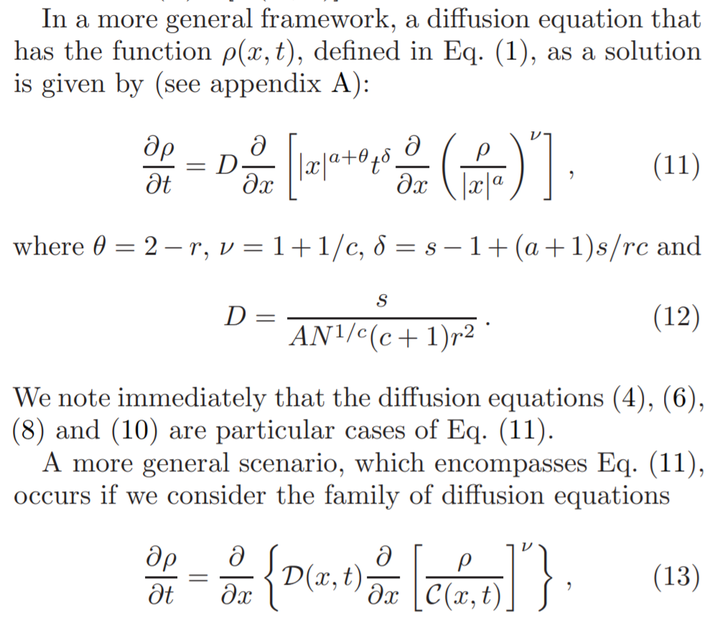# Stationary solution and H theorem for a generalized Fokker-Planck equation### Abstract

We investigate a family of generalized Fokker-Planck equations that contains Richardson and porous media equations as members. Considering a confining drift term that is related to an effective potential, we show that each equation of this family has a stationary solution that depends on this potential. This stationary solution encompasses several well-known probability distributions. Moreover, we verify an H theorem for the generalized Fokker-Planck equations using free-energy-like functionals. We show that the energy-like part of each functional is based on the effective potential and the entropy-like part is a generalized Tsallis entropic form, which has an unusual dependence on the position and can be related to a generalization of the Kullback-Leibler divergence. We also verify that the optimization of this entropic-like form subjected to convenient constraints recovers the stationary solution. The analysis presented here includes several studies about H theorems for other generalized Fokker-Planck equations as particular cases.

Type